# Atomic Model Terms

a) Atomic Number (Z): The atomic number of an element is the number of protons contained in the nucleus of the atom of that element.

b) Nucleons: Protons and neutrons are present in a nucleus. So, these fundamental particles are collectively known as nucleons.

c) Mass Number (A): The total number of protons and neutrons i.e, the number of nucleons present in the nucleus is called the mass number of the element.

d) Nuclide: Various species of atoms in general. A nuclide has specific value of atomic number and mass number.

IUPAC notation of an atom (nuclide)

Let X be the symbol of the element. Its atomic number be Z and mass number be A.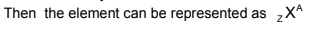e) Isotopes: Atoms of the element with the same atomic number but different mass number. There are three isotopes of hydrogen.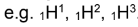f) Isobars: Atoms having the same mass number but different atomic numbers are called isobars.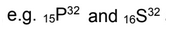g) Isotones: Atoms having the same number of neutrons but different number of protons or mass number are called isotones.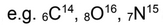h) Isoelectronic: Atoms, molecules or ions having the same number of electrons are isoelectronic.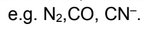i) Nuclear isomer: Nuclear isomers (isomeric nuclei) are the atoms with the same atomic number and the same mass number but with different radioactive properties.

Example of nuclear isomers is Uranium –X (half life 1.4 min) and Uranium –Z (half life 6.7 hours)

The reason for nuclear isomerism is the different energy states of the two isomeric nuclei.

Other examples are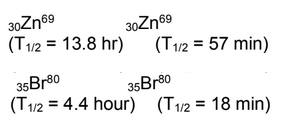j) Isosters: Molecules having same number of atoms and also the same number of electrons are called isosters.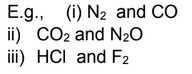k) Atomic mass unit: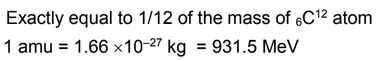Post By : Divya SIngh 27 Dec, 2019 3308 views Chemistry